Imagination and the Impossible

Two more sources I’d like to draw from for this fall’s maths for designers-course:

A fantastic collection of handouts for a two week summer workshop entitled ’Geometry and the Imagination’, led by John Conway, Peter Doyle, Jane Gilman and Bill Thurston at the Geometry Center in Minneapolis, June 1991, based on a course ‘Geometry and the Imagination’ they taught twice before at Princeton.

Among the goodies a long list of exercises in imagining (always useful to budding architects) and how to compute curvature by peeling potatoes and other vegetables…

The course really shines in giving a unified elegant classification of the 17 wallpaper groups, the 7 frieze groups and the 14 families of spherical groups by using Thurston’s concept of orbifolds.

If you think this will be too complicated, have a look at the proof that the orbifold Euler characteristic of any symmetry pattern in the plane with bounded fundamental domain is zero :

Take a large region in the plane that is topologically a disk (i.e. without holes). Its Euler characteristic is $1$. This is approximately equal to $N$ times the orbifold Euler characteristic for some large $N$, so the orbifold Euler characteristic must be $0$.

This then leads to the Orbifold Shop where they sell orbifold parts:

• a handle for 2 Euros,
• a mirror for 1 Euro,
• a cross-cap for 1 Euro,
• an order $n$ cone point for $(n-1)/n$ Euro,
• an order $n$ corner reflector for $(n-1)/2n$ Euro, if you have the required mirrors to install this piece.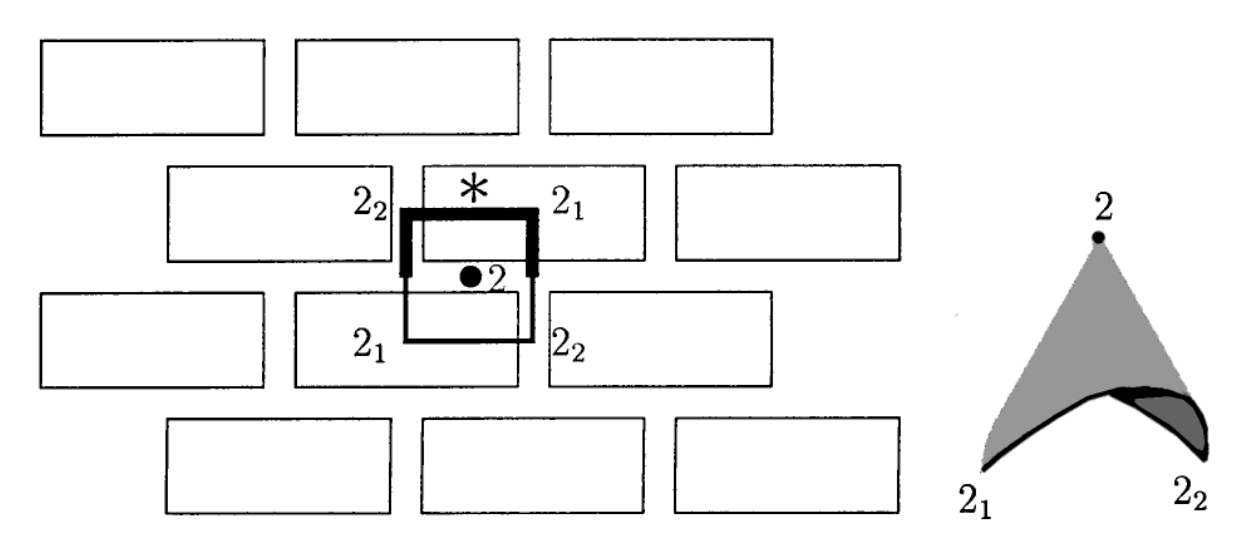Here’s a standard brick wall, with its fundamental domain and corresponding orbifold made from a mirror piece (1 Euro), two order $2$ corner reflectors (each worth $.25$ Euro), and one order $2$ cone point (worth $.5$ Euro). That is, this orbifold will cost you exactly $2$ Euros.

If you spend exactly $2$ Euros at the Orbifold Shop (and there are $17$ different ways to do this), you will have an orbifold coming from a symmetry pattern in the plane with bounded fundamental domain, that is, one of the $17$ wallpaper patterns.

For the mathematicians among you desiring more details, please read The orbifold notation for two-dimensional groups by Conway and Daniel Huson, from which the above picture was taken.

The aspiring architect should be warned that some constructions are simply not possible in 3D, even when they look convincing on paper, such as Escher’s Waterfall.M.C. Escher, Waterfall – Photo Credit

In his paper, Penrose gives a unified approach to debunk such drawings by using cohomology groups.

Clearly I have no desire to introduce cohomology, but it may still be possible to get the underlying idea across. Let’s take the Penrose triangle (all pictures below taken from Penrose’s paper)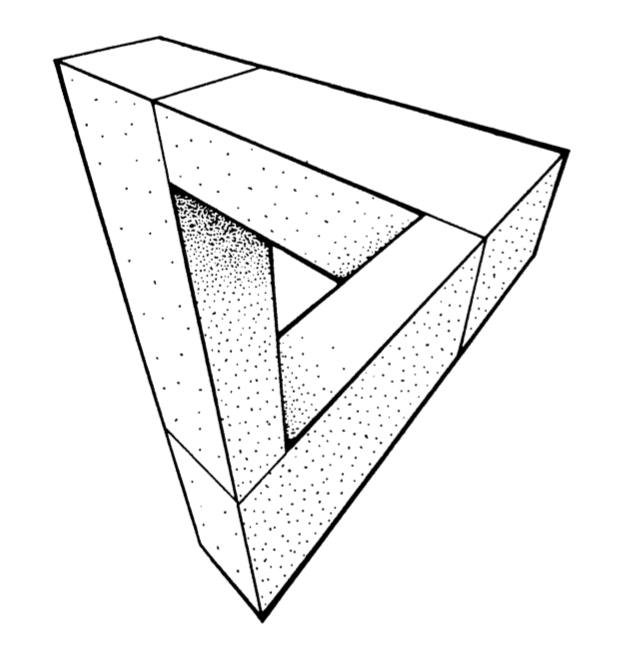The idea is to break up such a picture in several parts, each of which we do know to construct in 3D (that is, we take a particular cover of our figure). We can slice up the Penrose triangle in three parts, and if you ever played with Lego you’ll know how to construct each one of them.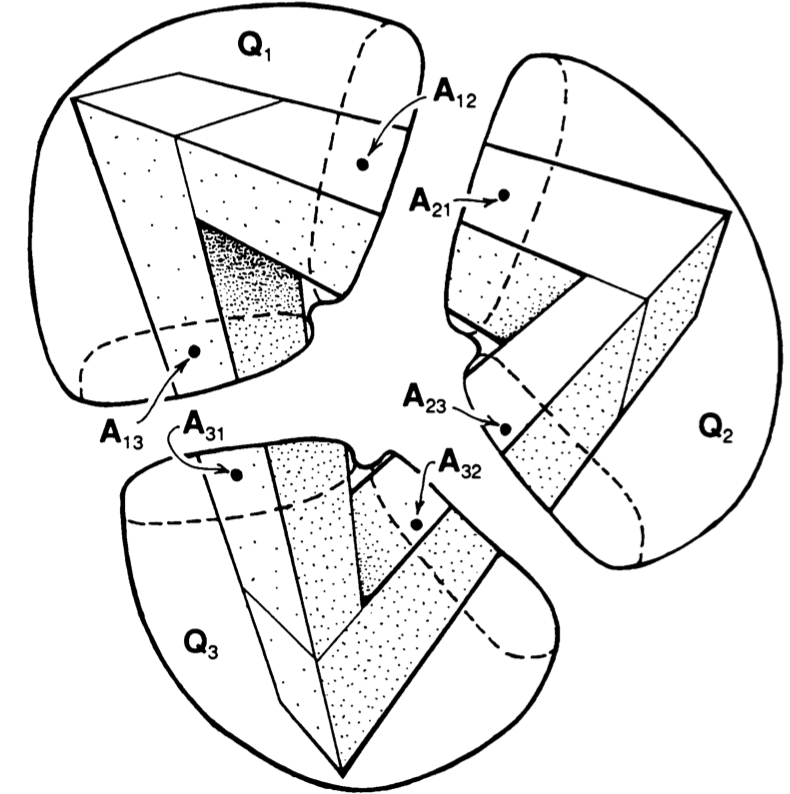Next, position the constructed pieces in space as in the picture and decide which of the two ends is closer to you. In $Q_1$ it is clear that point $A_{12}$ is closer to you than $A_{13}$, so we write $A_{12} < A_{13}$.

Similarly, looking at $Q_2$ and $Q_3$ we see that $A_{23} < A_{21}$ and that $A_{31} < A_{32}$.

Next, if we try to reassemble our figure we must glue $A_{12}$ to $A_{21}$, that is $A_{12}=A_{21}$, and similarly $A_{23}=A_{32}$ and $A_{31}=A_{13}$. But, then we get
$A_{13}=A_{31} < A_{32}=A_{23} < A_{21}=A_{12} < A_{13}$ which is clearly absurd.

Once again, if you have suggestions for more material to be included, please let me know.

Escher’s stairs

Stairways feature prominently in several drawings by Maurits Cornelis (“Mauk”) Escher, for example in this lithograph print Relativity from 1953.Relativity (M. C. Escher) – Photo Credit

In the world of ‘Relativity’, there are three sources of gravity, each being orthogonal to the two others.
Each inhabitant lives in one of the gravity wells, where normal physical laws apply.
There are sixteen characters, spread between each gravity source, six in one and five each in the other two.
The apparent confusion of the lithograph print comes from the fact that the three gravity sources are depicted in the same space.
The structure has seven stairways, and each stairway can be used by people who belong to two different gravity sources.

Escher’s inspiration for “Relativity” (h/t Gerard Westendorp on Twitter) were his recollections of the staircases in his old secondary school in Arnhem, the Lorentz HBS.
The name comes from the Dutch physicist and Nobel prize winner Hendrik Antoon Lorentz who attended from 1866 to 1869, the “Hogere Burger School” in Arnhem, then at a different location (Willemsplein).Stairways Lorentz HBS in Arnhem – Photo Credit

Between 1912 and 1918 Mauk Escher attended the Arnhem HBS, located in the Schoolstraat and build in 1904-05 by the architect Gerrit Versteeg. The school building is constructed around a monumental central stairway.Arnhem HBS – G. Versteeg 1904-05 – Photo CreditPlan HBS-Arnhem by G. Versteeg – Photo Credit

If you flip the picture below in the vertical direction, the two side-stairways become accessible to figures living in an opposite gravitation field.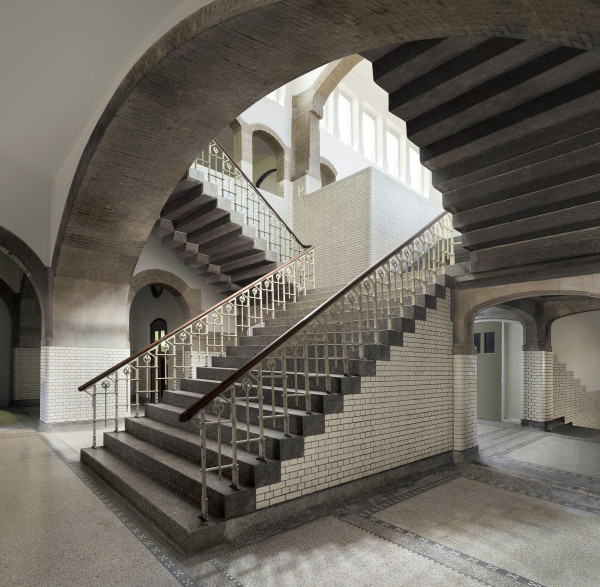Central staircase HBS Arnhem – Photo Credit

There’s an excellent post on the Arnhem-years of Mauk Escher by Pieter van der Kuil. Unfortunately (for most of you) in Dutch, but perhaps Google translate can do its magic here.

Coxeter on Escher’s Circle Limits

Conway’s orbifold notation gives a uniform notation for all discrete groups of isometries of the sphere, the Euclidian plane as well as the hyperbolic plane.

This includes the groups of symmetries of Escher’s Circle Limit drawings. Here’s Circle Limit III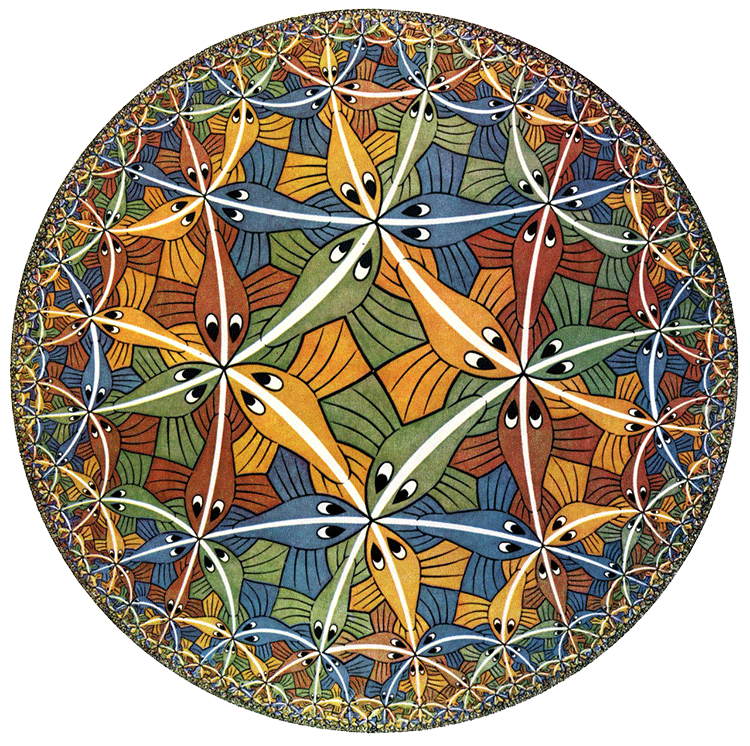And ‘Angels and Devils’ aka Circle Limit IV: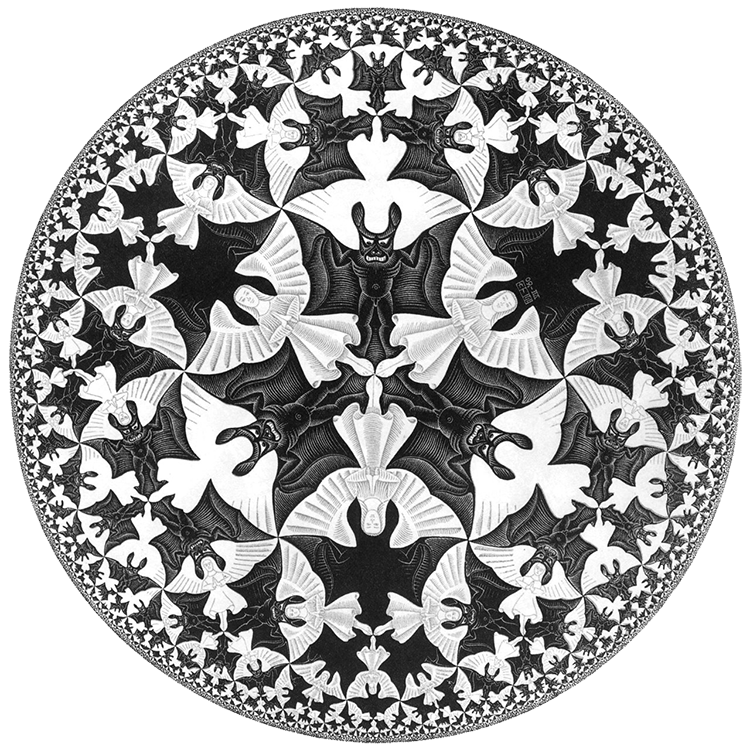If one crawls along a mirror of this pattern until one hits another mirror and then turns right along this mirror and continues like this, you get a quadrilateral path with four corners $\frac{\pi}{3}$, whose center seems to be a $4$-fold gyration point. So, it appears to have symmetry $4 \ast 3$.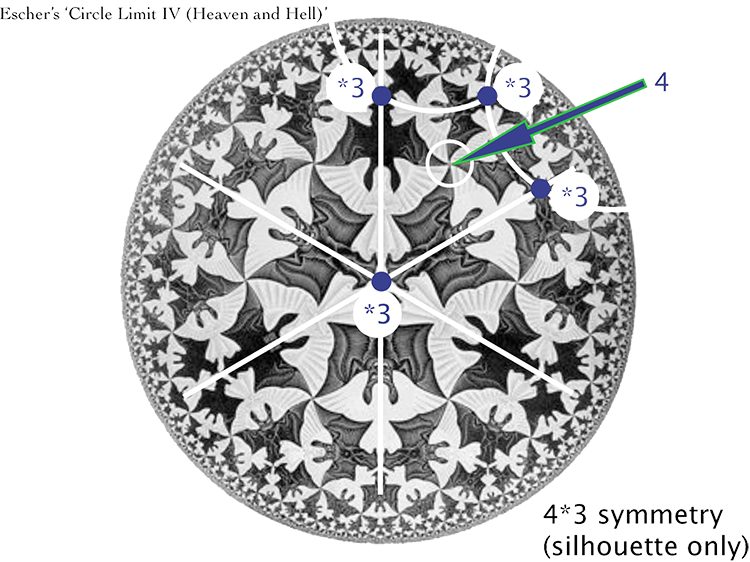(image credit: MathCryst)

However, looking more closely, every fourth figure (either devil or angel) is facing away rather than towards us, so there’s no gyration point, and the group drops to $\ast 3333$.

Harold S. M. Coxeter met Escher in Amsterdam at the ICM 1954.

The interaction between the two led to Escher’s construction of the Circle Limits, see How did Escher do it?

Here’s an old lecture by Coxeter on the symmetry of the Circle Limits: